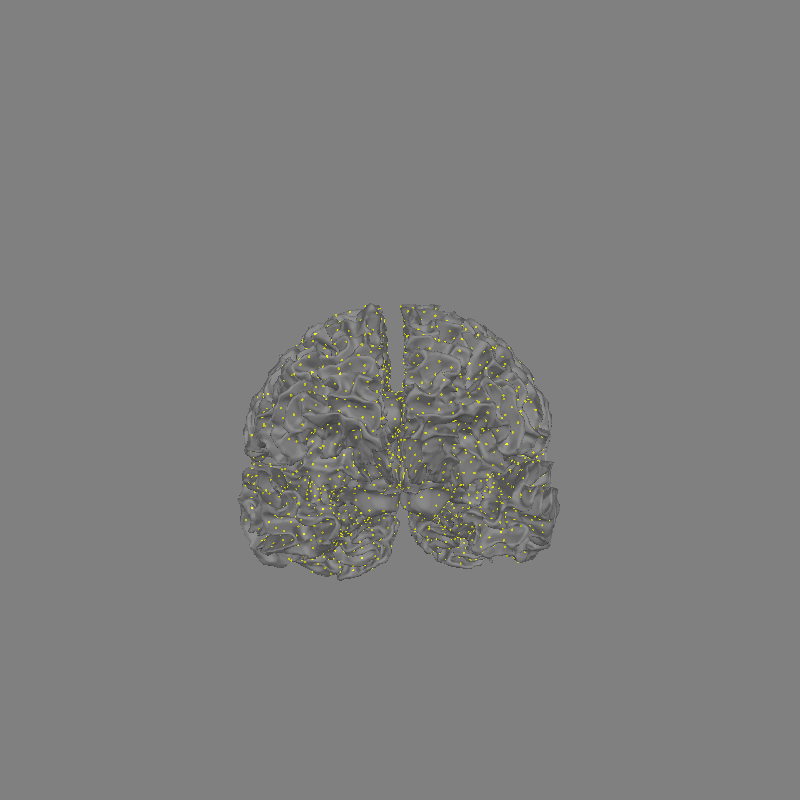The inverse operator’s source space is shown in 3D.

```# Author: Alexandre Gramfort <alexandre.gramfort@inria.fr>
#
```
```import mne
from mne.datasets import sample
from mne.viz import set_3d_view

print(__doc__)

data_path = sample.data_path()
subjects_dir = data_path / "subjects"
meg_path = data_path / "MEG" / "sample"
fname_trans = meg_path / "sample_audvis_raw-trans.fif"
inv_fname = meg_path / "sample_audvis-meg-oct-6-meg-inv.fif"

print("Method: %s" % inv["methods"])
print("fMRI prior: %s" % inv["fmri_prior"])
print("Number of sources: %s" % inv["nsource"])
print("Number of channels: %s" % inv["nchan"])

src = inv["src"]  # get the source space

print("Number of vertices on the left hemisphere: %d" % len(src["rr"]))
print("Number of triangles on left hemisphere: %d" % len(src["use_tris"]))
print("Number of vertices on the right hemisphere: %d" % len(src["rr"]))
print("Number of triangles on right hemisphere: %d" % len(src["use_tris"]))
```
```Reading inverse operator decomposition from /home/circleci/mne_data/MNE-sample-data/MEG/sample/sample_audvis-meg-oct-6-meg-inv.fif...
[done]
[done]
305 x 305 full covariance (kind = 1) found.
Read a total of 4 projection items:
PCA-v1 (1 x 102) active
PCA-v2 (1 x 102) active
PCA-v3 (1 x 102) active
Average EEG reference (1 x 60) active
22494 x 22494 diagonal covariance (kind = 2) found.
22494 x 22494 diagonal covariance (kind = 6) found.
22494 x 22494 diagonal covariance (kind = 5) found.
Did not find the desired covariance matrix (kind = 3)
Computing patch statistics...
[done]
Computing patch statistics...
[done]
Read a total of 4 projection items:
PCA-v1 (1 x 102) active
PCA-v2 (1 x 102) active
PCA-v3 (1 x 102) active
Average EEG reference (1 x 60) active
Source spaces transformed to the inverse solution coordinate frame
Method: 1
fMRI prior: None
Number of sources: 7498
Number of channels: 305
Number of vertices on the left hemisphere: 155407
Number of triangles on left hemisphere: 8192
Number of vertices on the right hemisphere: 156866
Number of triangles on right hemisphere: 8192
```

Show the 3D source space

```fig = mne.viz.plot_alignment(
subject="sample",
subjects_dir=subjects_dir,
trans=fname_trans,
surfaces="white",
src=src,
)
set_3d_view(fig, focalpoint=(0.0, 0.0, 0.06))
```Total running time of the script: (0 minutes 4.809 seconds)

Estimated memory usage: 26 MB

Gallery generated by Sphinx-Gallery